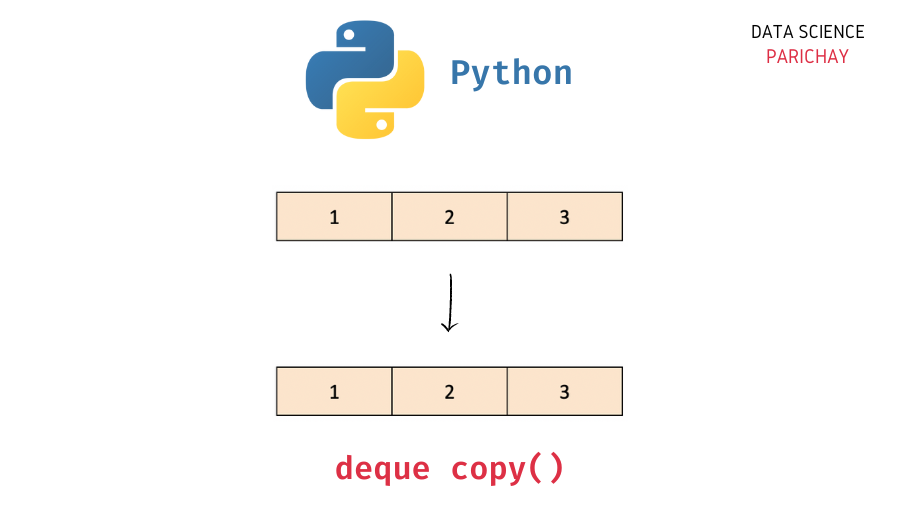# Python – Create a deque copy

In this tutorial, we will look at how to copy a deque in Python with the help of some examples.

## `deque` in Python

The `collections` module in Python comes with a `deque` class that you can use to implement a stack or queue data structure in Python. Deques support thread-safe, memory efficient appends and pops from either side of the deque with approximately the same O(1) performance in either direction.

In simple terms, you can think of a `deque` in Python as a list-like data structure but with efficient insertion and removal of items from either end (left and right). This makes them very useful when implementing a stack or queue functionality in Python.

## How to copy a deque in Python?

The `deque` class comes with a number of built-in functions to help perform common operations (like adding and removing elements).

To create a copy of a deque object in Python, use the deque `copy()` function. The following is the syntax –

```# create copy of deque "queue"
queue.copy()```

It creates a shallow copy of the deque and returns it.

## Example – Create a copy of a deque object

Let’s now look at some examples of using the above syntax –

First, let’s create a deque with some elements.

📚 Data Science Programs By Skill Level

Introductory

Intermediate ⭐⭐⭐

🔎 Find Data Science Programs 👨‍💻 111,889 already enrolled

Disclaimer: Data Science Parichay is reader supported. When you purchase a course through a link on this site, we may earn a small commission at no additional cost to you. Earned commissions help support this website and its team of writers.

```from collections import deque

# create a deque
queue = deque([1, 2, 3])
# print the deque
print(queue)```

Output:

`deque([1, 2, 3])`

Here, we imported the `deque` class from the `collections` module and created a deque object, `queue` with three elements – 1, 2, and 3.

Now, let’s now create a copy of the deque object created above.

```# create deque copy
queue_cp = queue.copy()
# display the returned deque
print(queue_cp)```

Output:

`deque([1, 2, 3])`

Here, we used the deque `copy()` function to create a copy of the deque `queue` and stored the resulting deque in the variable `queue_cp`.

Keep in mind that the `copy()` function creates a shallow copy. This means that the new deque object stores references to the values in the original deque object and does not have its own separate copy of each object from the original deque (like you would in the case of a deep copy).

This might not be an issue if you’re working with immutable types, for example, a deque of numbers.

```# modify queue_cp
queue_cp.append(4)
# display the copied deque
print(queue_cp)
# display the original deque
print(queue)```

Output:

```deque([1, 2, 3, 4])
deque([1, 2, 3])```

You can see that modifying the copied deque does not affect the original deque here.

But, if the deque stores mutable compound objects (like a list), making changes to the copied deque can affect the original deque, and vice-versa (since we’re working with a shallow copy here).

Let’s look at an example.

```# create a deque
queue = deque([1, 2, [3, 4]])
# create deque copy
queue_cp = queue.copy()
# display the returned deque
print(queue_cp)```

Output:

`deque([1, 2, [3, 4]])`

Here, we created a deque containing a list of numbers as one of its elements and then used the deque `copy()` function to create its copy.

Now, let’s modify the copied deque by adding an element to the list inside the deque.

```# modify queue_cp
queue_cp.append(5)
# display the copied deque
print(queue_cp)
# display the original deque
print(queue)```

Output:

```deque([1, 2, [3, 4, 5]])
deque([1, 2, [3, 4, 5]])```

You can see that both the copied and the original deques were modified because we made changes to a shallow copy (which does not have a separate copy of the values and rather works with references to the items in the original object).

You might also be interested in –

•